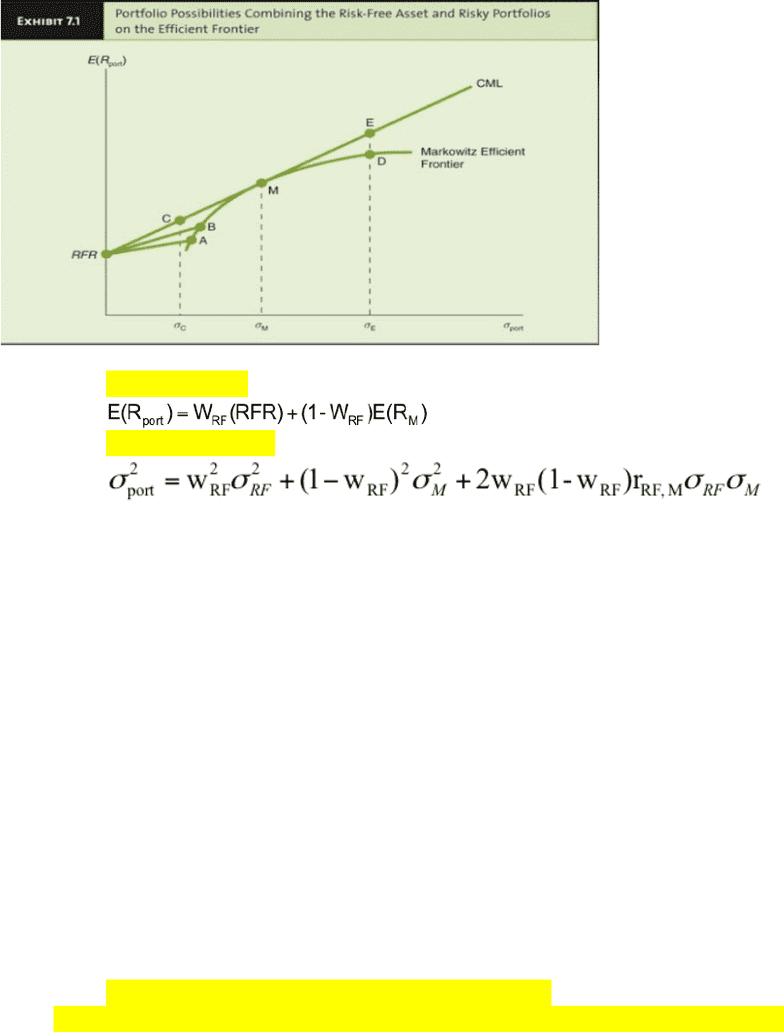Textbook Notes (280,000)
CA (170,000)
WLU (9,000)
BU (2,000)
BU473 (6)
Chapter 7

# BU473 Chapter 7: Chapter 7

Department
Course Code
BU473
Professor
Steve Balaban
Chapter
7

This preview shows page 1. to view the full 4 pages of the document.Chapter(7:(Asset(Pricing(Models(
Risk-Return+Possibilities++
Developing+a+Capital+Market+Line+
+
Combining+a+risk-free+asset+with+a+risky+portfolio,+M+
o Expected(return+=+the+weighted+average+of+the+2+assets++
o +
o Standard(deviation+=+applying+the+2-asset+standard+deviation+formula:++
o +
++
Concept+of+Risk-Free+Asset++
An+asset+with+zero+standard+deviation++
Zero+correlation+with+all+other+risky+assets++
Provides+the+risk-free+rate+of+return++
Will+lie+on+the+vertical+axis+of+a+portfolio+graph++
++
Risk,+Diversification+&+the+Market+Portfolio+++
Systematic+Risk++
Only+systematic+risk+remains+in+the+market+portfolio++
Firm-specific+risk++
o Growth+of+money+supply+variability+(macroeconomic)+
o Interest+rate+volatility++
o Variability+in+factors+like:++
Industrial+production++
Corporate+earnings++
Cash+flow++
o Can(be(measured(by(standard(deviation(of(returns+and+can+change+over+time++
Purpose(of(diversification(is(to(reduce(the(standard(deviation(of(the(total(portfolio(+
Assumes+that+imperfect+correlations+exist+among+securities++